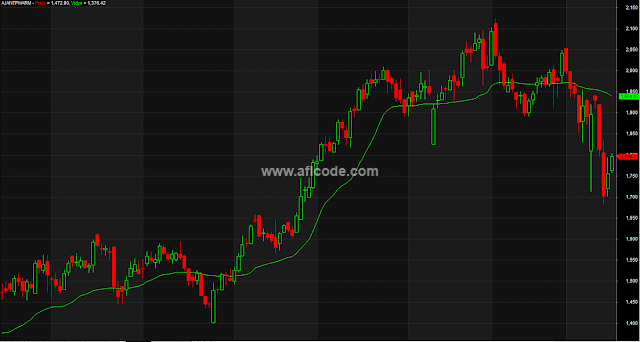### Magic Moving Average LineMagic Moving Average Line

```//www.aflcode.com
Length=21;
Smooth=5;

Change = C - Ref( C, -1 );
SU = Sum( C - Ref( C, -1 ) > 0, Length );
SD = Sum( C - Ref( C, -1 ) < 0, Length );
CMO = 100 * ( SU - SD ) / ( SU + SD );

AbsCMO=( abs(CMO) )/100;

SC=2/(Smooth+1);

VIDYA=AMA( C, SC*AbsCMO );

Plot( Close, "Price", 4, 64 );
Plot( VIDYA, "Vidya", 5 );
```
Previous
Next Post »